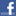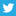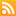Scott Hanselman

Top subtle as a brick in the face issues when converting my Tiny OS from C to VBNET Array LengthsI knew

October 08, 2002 Comment on this post  Posted in Web Services

Top subtle (as a brick in the face) issues when converting my Tiny OS from C# to VB.NET:

1. Array Lengths...I knew we were warned, and there were all those arguments from Beta1 to Beta2 to RTM, but still...

byte[] bytes = new byte;

has length 4, from 0 to 3

Dim bytes(4) As Byte

has length 5, from 0 to 4

2. Integer Divison... "/" and "\" are different operators in VB.NET than C#.  "/" doesn't round, while "\" does...

(uint)(boundary * ((number / boundary) + ((number % boundary > 0) ? 1: 0)))

where boundary is 16 and number is 82 returns 96.  While "equivalent (not)" VB.NET

CType(boundary * ((number / boundary) + IIf(number Mod boundary > 0, 1, 0)), Integer)

where boundary is 16 and number is 82 returns 98 because (number / boundary) returns 5.25, not 5.  This was fixed by using a backslash.

CType(boundary * ((number \ (BACKSLASH) boundary) + IIf(number Mod boundary > 0, 1, 0)), Integer)

This is one of these obvious, silly things you've known since VB3, but you don't think about it when converting from C# to VB.NET.

3. UInt32 isn't supported in VB, so I had to wimp out and switch to Integers.